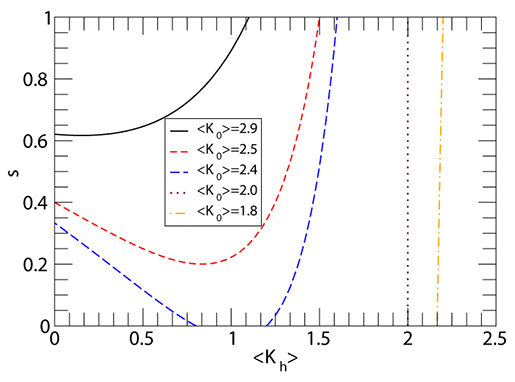Critical Phenomena
Through years, Statistical Physics has developed both theoretical and numerical methods that have proved to be powerful techniques in the study and understanding of many diverse fields of science. In this sense, the mathematical characterization of the problems and models to be explored is particularly difficult as they usually deal with non-equilibrium processes for which a theoretical framework similar to equilibrium statistical mechanics is not available. In this sense, computer simulations represent an essential method in the Physics of complex networks and systems. At the same time, they are invaluable tools to design conceptual experiments, which use the computer as a test field. Additionally, they allow us to explore different theoretical alternatives that include methods such as: dynamical mean field calculations, renormalization group schemes and other techniques drawn from the study of critical phenomena. The are different numerical and analytical methods to achieve this goal:

1) Numerical methods:
1. Statistical characterization of real complex networks.
2. Development and numerical exploration of network models.
3. Numerical simulations of dynamical processes on complex networks.
2) Analytical methods:
1. Dynamical mean-feld theories: This formalism allows studying the behavior of dynamical systems defined on the underlying topology of complex networks.
2. Percolative and probabilistic methods: The use of some percolative and probabilistic tools seems to be a good starting point to study analytically some kind of models and dynamical processes on complex networks. This is the case, for instance, of generating function techniques and of models than can be cast into autonomous differential systems such as some ecological problems and models of infection, rumor or information spreading.
3. Renormalization group: The goal of this method is to find the RG equations that allow the computation of scaling exponents and the discovery of universality classes. This could be achieved by studying the behavior of some observable quantity under the integration of degrees of freedom, for example, by merging similar neighboring nodes.

Development of analytical tools to study complex networks

1. Application of dynamical mean-field techniques to analytically study the effects of networks’ heterogeneity in the behavior of dynamical systems defined on them.
2. Use of scaling arguments to predict the behavior of finite-size real networks.
3. Application of percolation theory approaches and generating function techniques to obtain real quantities such as critical points and average values of observable quantities.
4.Find out more by checking our main publications at: MAIN PUBLICATIONS